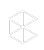Learn from the best and brightest in legal, technology, and beyond! Join us at the 2019 Clio Cloud Conference — San Diego | October 21-22, 2019.

# When Will Interest Start Accruing?

Objective:

• When will I incur my bill's first interest charge?
• Applying Interest to Bills
• When will I first be charged interest?
• Payment Profile in practice samples

Environment:

• Clio Web App

Additional Information:

For information on when interest is applied to an invoice, see here.

For how to apply interest on an already outstanding bill, see here

Answer:

• Grace period: This will automatically push the due date (X) days
• Interest will always appear a day after the due date as well as every (Y) days from the issue date (due to the interest interval)

Full Explanation (with examples!)

• Interest is first calculated based on the timespan between the bill's issue date and due date. The initial interest charge will appear once the bill is approved and the due date has passed (one day after the due date). After the initial charge, subsequent charges will depend on the interest interval.

• For example, if the due date is set to be 15 days after the issue date and the interest interval is 30 days; You will see the initial charge on day 16 (day after the expired due date) and then subsequent charges on days 46, 76, etc (due to the interest interval)

• The grace period serves as a way of automatically setting the due date (X) days from the issue date.
• For example, if the grace period is 25 days, set to accrue interest every 30 days; You will see an interest charge on day 26 (for the expired grace period/due date of 25 days), and again on day 56 (due to the interest interval).

• If the grace period and the interest interval are the same (X) then the initial interest charge will occur one day after the expiration of the due date ((X) days after the issue date) and subsequent charges every (X) days.
• For example, if the period is a 20 day grace period, set to accrue interest every 20 days; You will see an interest charge on day 21 (for the expired grace period/due date), and again on day 41 (due to the interest interval).

• If the grace period (X) is more than the interest interval (Y) then the initial interest charge will occur one day after the expiration of the due date ((X) days after the issue date) and subsequent charges every (Y) days.
• For example, if the period is a 30 day grace period, set to accrue interest every 15 days; You will see an interest charge on day 31 (for the expired grace period/due date), and again on day 46 (due to the interest interval).

• If there is no grace period, then the first interest charge will occur the day of the invoice plus the interest interval.

• For example, if a payment profile has a 0 day Grace Period, and interest is charged every 30 days; You will see the first interest charge on the 31st day, and it will include charges covering the first 31 days.
• If you are backdating a Bill, it can take up to 24 hours for the interest to apply.
Was this article helpful?
This information is confusing or wrong
This isn't the information that I was looking for
I don't like this functionality